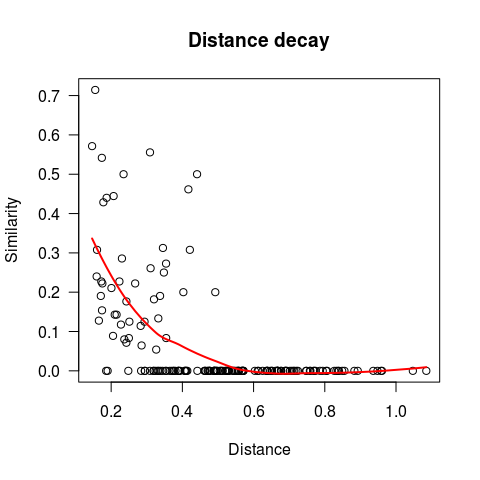# Analysis of biodiversity patterns

#### 2017-11-02

This introduction shows the list of biodiversity patterns that can be derived using mobsim. In this vignette the patterns are evaluated on a simulated data set, but of course the same patterns can be also derived from real data.

# 1 Simulate and explore data

First, we generate community data by simulating 1,000 individuals from 30 species with a cluster size of sigma = 0.01 and one cluster per species (mother_points = 1). For more information on the simulation of communities see the vignette Simulating communities with mobsim.

library(mobsim)
sim_dat1 <- sim_thomas_community(s_pool = 30,  n_sim = 1000, sad_type = "lnorm",
sad_coef = list("meanlog" = 2, "sdlog" = 1),
sigma = 0.1, mother_points = 1)

Then we explore the generated community object. In the plot each dot represents one individual and the colour indicates the species identity.

plot(sim_dat1)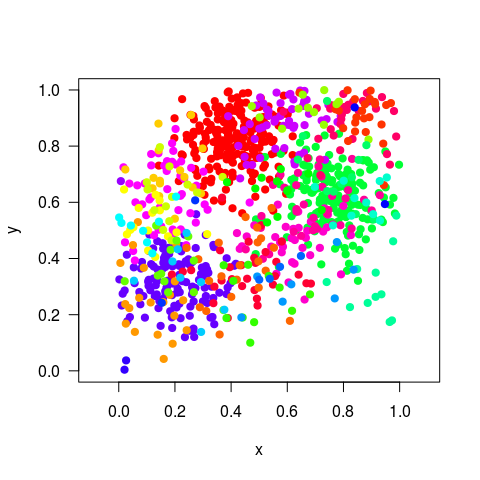summary(sim_dat1)
## No. of individuals:  1000
## No. of species:  30
## x-extent:  0 1
## y-extent:  0 1
##
##        x                 y                species
##  Min.   :0.00139   Min.   :0.004044   species1:220
##  1st Qu.:0.26755   1st Qu.:0.437298   species2:194
##  Median :0.48398   Median :0.638965   species3:135
##  Mean   :0.48862   Mean   :0.613282   species4: 54
##  3rd Qu.:0.71609   3rd Qu.:0.797226   species5: 46
##  Max.   :0.99783   Max.   :0.999437   species6: 36
##                                       (Other) :315
str(sim_dat1)
## List of 3
##  $census :'data.frame': 1000 obs. of 3 variables: ## ..$ x      : num [1:1000] 0.405 0.477 0.533 0.443 0.35 ...
##   ..$y : num [1:1000] 0.795 0.786 0.941 0.806 0.811 ... ## ..$ species: Factor w/ 30 levels "species1","species10",..: 1 1 1 1 1 1 1 1 1 1 ...
##  $x_min_max: num [1:2] 0 1 ##$ y_min_max: num [1:2] 0 1
##  - attr(*, "class")= chr "community"

# 2 Non-spatial patterns

## 2.1 Species abundance distribution (SAD)

A fundamental non-spatial pattern of a community is the abundance distribution, i.e. the distribution of commonness and rarity in a community. The abundance distribution can be extracted from a community object using the function communtiy_to_sad()

abund1 <- community_to_sad(sim_dat1)

A standard plot in community ecology is the rank-abundance plot, where the abundance of each species is plotted vs. its rank from highest to lowest abundance. As a standard log-scaling is used for the abundance axis in this plot.

plot(abund1, method = "rank")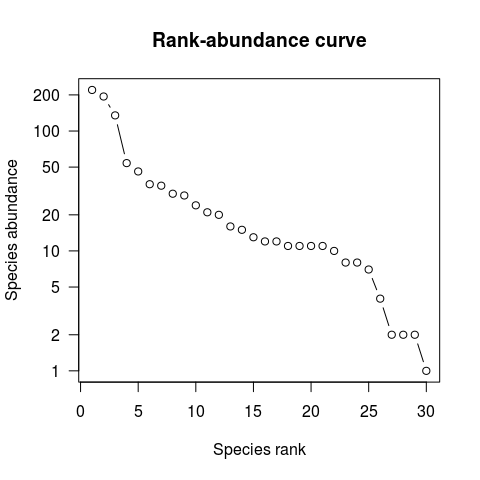Of course the abundance distribution can be also visualized as a histogram. By tradition for the binning of species abundances logarithms with base 2 are used, following the suggestion of Preston (1948). This means the first abundance class includes species with just one individual, the second class with two individuals, the third class with 3-4, the fourth with 5-8 etc. These abundance classes are called “octaves”.

plot(abund1, method = "octave")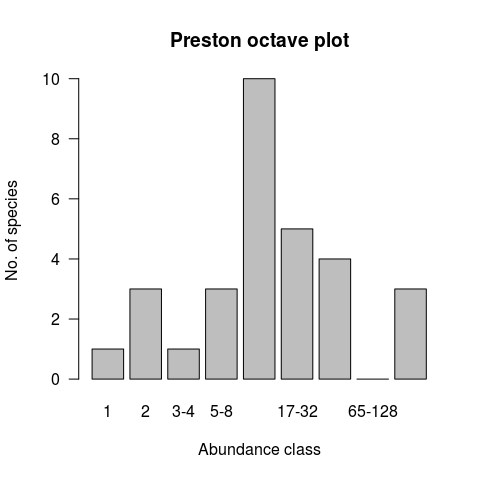## 2.2 Rarefaction curve

Another important biodiversity pattern is the rarefaction curve, which estimates how the number of observed species increases with sample size of individuals. The rarefaction curve assumes that individuals are sampled randomly and independently (Gotelli & Colwell 2001). The rarefaction curve only depends on the abundance distribution of species.

rare1 <- spec_sample_curve(sim_dat1, method = "rarefaction")
str(rare1)
## Classes 'spec_sample_curve' and 'data.frame':    1000 obs. of  2 variables:
##  $n : int 1 2 3 4 5 6 7 8 9 10 ... ##$ spec_rarefied: num  1 1.88 2.67 3.38 4.03 ...
plot(rare1)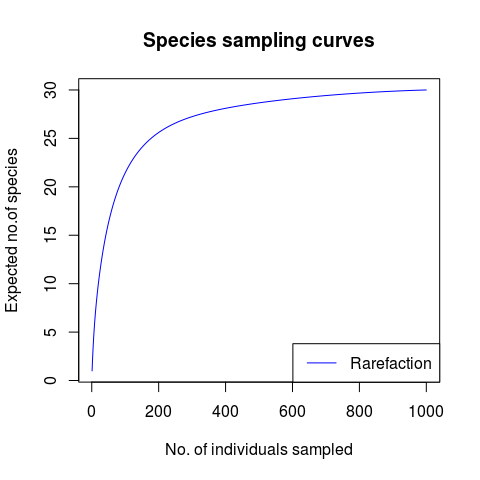# 3 Spatial patterns

The main strengths of mobsim are the simulation and analysis of spatial biodiversity patterns. In the following all functions for spatial pattern evaluation included in mobsim are introduced.

## 3.1 Species-accumulation curve

Closely related to the rarefaction curve is the spatial species accumulation curve. In contrast to the rarefaction curve for the derivation of the species accumulation curve individuals are not sampled randomly, but starting from a focal individual always the closest neighbour individual is sampled and the number of encountered species is counted. The resulting curve is derived as the average of the curves for each focal individual. The species accumulation curve is influenced by the abundance distribution, but also by the spatial distribution of individuals and species. Therefore the species accumulation curves requires individual’s positions in addition to species abundances.

Due to the close relationship of the accumulation and rarefaction curves they are calculated and plotted with the same function.

It is comprehensive to plot the rarefaction and the species accumulation curve together. The difference between the two curves indicates aggregation or overdispersion of conspecific individuals.

spec_curves1 <- spec_sample_curve(sim_dat1, method = c("accumulation", "rarefaction"))
plot(spec_curves1)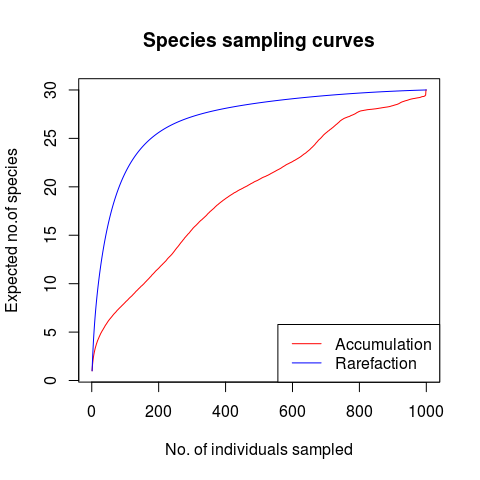## 3.2 Diversity-area relationships

The most well-known spatial biodiversity pattern is the species-area relationship (SAR). In mobsim the function divar (diversity-area relationships) calculates the species richness in randomly located subplots (quadrats or rectangles) of different sizes. However, the function divar calculates additional indices, including the Shannon and Simpson diversity indices for each subplot, as well as the number of endemic species, which are the species that only occur within, but not outside the subplot. See ?div_rect for detailed information on the diversity indices.

For the Shannon and Simpson diversity indices also the corresponding Effective Number of Species (ENS) is calculated (Jost 2006). This measure corresponds to the species number in a community of equally abundant species, which results in the same Shannon and Simpson indices as the observed community (with unequal abundances).

The endemics-area relationship (EAR) has been suggested as important tool to investigate the consequences of habitat loss for biodiversity (He & Hubbell 2011, Keil et al. 2015). For the evaluation of the diversity-area relationships a vector with subplot sizes measured as proportion of the total community size has to be defined.

subplot_size <- c(0.01, 0.05, 0.1, 0.2, 0.3, 0.4, 0.5,
0.6, 0.7, 0.8, 0.9, 0.95, 0.99, 1)
divar1 <- divar(sim_dat1, prop_area = subplot_size)
head(divar1)
##   prop_area m_species sd_species m_endemics sd_endemics m_shannon
## 1      0.01  3.340909   1.522901       0.00   0.0000000 0.8468817
## 2      0.05  7.300000   2.329000       0.02   0.1407053 1.3801689
## 3      0.10 10.690000   2.743937       0.08   0.2726599 1.6301731
## 4      0.20 15.650000   3.002945       0.66   0.7278028 1.9339171
## 5      0.30 19.910000   2.099038       1.83   1.0546195 2.1519223
## 6      0.40 23.680000   1.774568       3.40   1.4770979 2.3263989
##   sd_shannon m_ens_shannon sd_ens_shannon m_simpson sd_simpson
## 1  0.4863798      2.610717       1.248707 0.5078010 0.20659544
## 2  0.4797379      4.413384       1.902684 0.6278702 0.19290688
## 3  0.4158965      5.539084       2.201456 0.6893783 0.15318832
## 4  0.2806888      7.181811       1.937657 0.7584420 0.09544478
## 5  0.1877812      8.754716       1.676637 0.8001405 0.04722183
## 6  0.1363897     10.335534       1.406726 0.8382316 0.02871204
##   m_ens_simpson sd_ens_simpson
## 1      2.436673       1.117590
## 2      3.454685       1.673279
## 3      4.066093       1.931898
## 4      4.775628       1.748823
## 5      5.308201       1.335871
## 6      6.378798       1.137760
plot(divar1)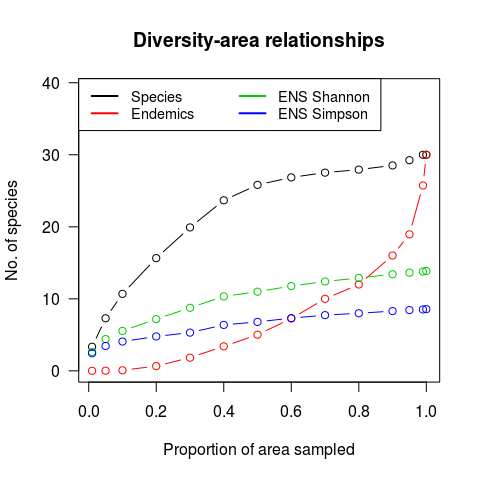## 3.3 Distance decay of community similarity

The last spatial pattern provided by mobsim is the distance decay of community similarity, which quantifies how quickly the similarity in species composition between two subplots decreases with the distance between two subplots. The function dist_decay distributes non-overlapping subplots with user-defined size and number in the community and calculates all pairwise similarities.

The function dist_decay makes use of the function vegdist from the package vegan. Therefore you can consult ?vegdist for a list of available similarity indices.

Here is a demonstration how the distance decay can be estimated and visualized

dd1 <- dist_decay(sim_dat1, prop_area = 0.01, n_samples = 20, method = "bray")
head(dd1)
##      distance similarity
## 84  0.1464524  0.5714286
## 110 0.1552740  0.7142857
## 77  0.1592714  0.2400000
## 17  0.1603262  0.3076923
## 149 0.1655349  0.1276596
## 75  0.1709832  0.1904762
plot(dd1)